﻿ A Study of the S-Generalized Gauss Hypergeometric Function and Its Associated Integral Transforms

### A Study of the S-Generalized Gauss Hypergeometric Function and Its Associated Integral Transforms

H. M. Srivastava, Rashmi Jain, M. K. Bansal

Turkish Journal of Analysis and Number Theory

## A Study of the S-Generalized Gauss Hypergeometric Function and Its Associated Integral Transforms

H. M. Srivastava1, 2,, Rashmi Jain3, M. K. Bansal3

1Department of Mathematics and Statistics, University of Victoria, Victoria, British Columbia V8W 3R4, Canada

2China Medical University, Taichung 40402, Taiwan, Republic of China

3Department of Mathematics, Malaviya National Institute of Technology, Jaipur 302017, Rajasthan, India

### Abstract

The aim of the present paper is to further investigate the S-generalized Gauss hypergeometric function which was recently introduced by Srivastava et al. . In the course of our study, we first present an integral representation, the Mellin transform and a complex integral representation of the S-generalized Gauss hypergeometric function. Next, we introduce a new integral transform whose kernel is the S-generalized Gauss hypergeometric function and point out its three special cases which are also believed to be new. We specify that the well-known Gauss hypergeometric function transform follows as a simple special case of our integral transforms. Finally, we establish an inversion formula for the integral transform which we have introduced in this investigation.

• H. M. Srivastava, Rashmi Jain, M. K. Bansal. A Study of the S-Generalized Gauss Hypergeometric Function and Its Associated Integral Transforms. Turkish Journal of Analysis and Number Theory. Vol. 3, No. 5, 2015, pp 116-119. http://pubs.sciepub.com/tjant/3/5/1
• Srivastava, H. M., Rashmi Jain, and M. K. Bansal. "A Study of the S-Generalized Gauss Hypergeometric Function and Its Associated Integral Transforms." Turkish Journal of Analysis and Number Theory 3.5 (2015): 116-119.
• Srivastava, H. M. , Jain, R. , & Bansal, M. K. (2015). A Study of the S-Generalized Gauss Hypergeometric Function and Its Associated Integral Transforms. Turkish Journal of Analysis and Number Theory, 3(5), 116-119.
• Srivastava, H. M., Rashmi Jain, and M. K. Bansal. "A Study of the S-Generalized Gauss Hypergeometric Function and Its Associated Integral Transforms." Turkish Journal of Analysis and Number Theory 3, no. 5 (2015): 116-119.

 Import into BibTeX Import into EndNote Import into RefMan Import into RefWorks

### 1. Introduction and Definitions

The S-generalized Gauss hypergeometric function: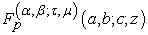was introduced and investigated by Srivastava et al. [, p. 350, Eq. (1.12)]. It is represented in the following manner: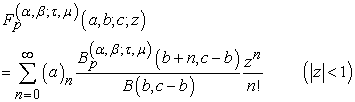(1.1)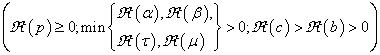in terms of the classical Beta function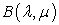and the S-generalized Beta function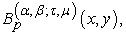which was also defined by Srivastava et al. [, p. 350, Eq. (1.13)] as follows: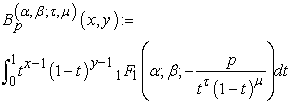(1.2)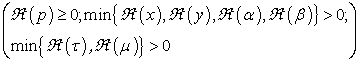and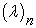denotes the Pochhammer symbol defined (for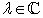) by (see [, p. 2 and pp. 4-6]; see also [, p. 2]):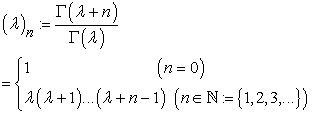(1.3)

provided that the Gamma quotient exists (see, for details, [, p. 16 et seq.] and [, p. 22 et seq.]).

For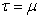, the S-generalized Gauss hypergeometric function defined by (1.1) reduces to the following generalized Gauss hypergeometric function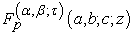studied earlier by Parmar [, p.44]: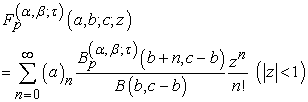(1.4)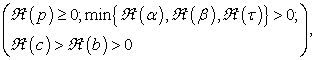which, in the further special case when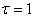, reduces to the following extension of the generalized Gauss hypergeometric function (see, e.g., [, p. 4606, Section 3]; see also [, p. 39]):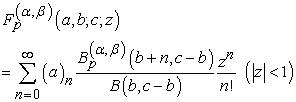(1.5)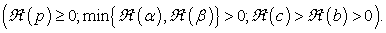Upon setting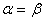in (1.5), we arrive at the following extended Gauss hypergeometric function (see [, p.591, Eqs. (2.1) and (2.2)]):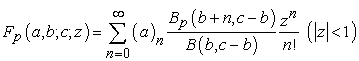(1.6)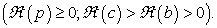In the present paper. we propose to further investigate the S-generalized Gauss hypergeometric function defined by (1.1). We first derive an integral representation, the Mellin transform and a complex integral representation of the S-generalized Gauss hypergeometric function. We also introduce and study a new integral transform whose kernel is the S-generalized Gauss hypergeometric function and point out its three special cases which are also believed to be new. The well-known Gauss hypergeometric function transform follows as a simple special case of our integral transforms. Finally, we establish an inversion formula for the integral transform which we have introduced in this investigation. For other related works on various families of Gauss and Kummer hypergeometric functions and their multi-parameter extensions and generalizations, one may refer (for example) to the recent papers [1, 3, 4] and .

### 2. A Set of Main Results

In this section, we first give the aforementioned integral representation, the Mellin Transform and a complex integral representation of the S-generalized Gauss hypergeometric function. We also introduce a new integral transform whose kernel is the S-generalized Gauss hypergeometric function defined by (1.1).

2.1. Integral Representation of the S-Generalized Gauss Hypergeometric Function

Theorem 1. Suppose that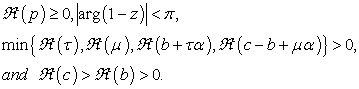Then the following integral representation holds true: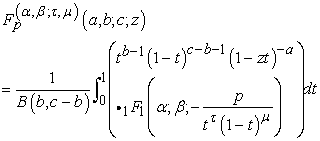(2.1)

where the S-generalized Gauss hypergeometric functionis given by (1.1).

Proof. Using Eq. (1.1) on the left-hand side of (2.1), we find that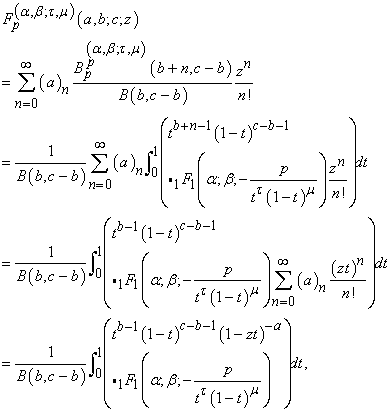which proves Theorem 1.

2.2. The Mellin Transform of the S-Generalized Gauss Hypergeometric Function

As usual, the Mellin transform of a function f(t) is defined by (see, for example, [, p. 340, Eq. (8.2.5)])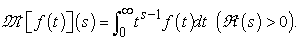(2.2)

provided that the improper integral exists.

Theorem 2. If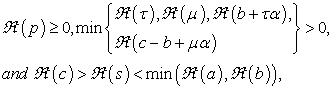then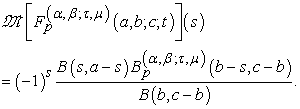(2.3)

Proof. In order to prove the assertion (2.3), by taking the Mellin transform of (2.1), we obtain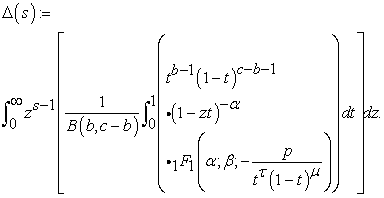Upon interchanging the order of the t- and the z-integrals (which is permissible under the conditions stated), if we evaluate the resulting z-integral first, we get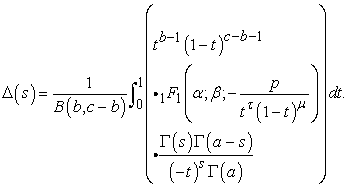Now, with the help of (1.2), we get the desired result (2.3) after a little simplification.

2.3. A Complex Integral Representation of the S-Generalized Gauss Hypergeometric Function

If we take the inverse Mellin transform of (2.3), we easily arrive at the following complex integral representation for the S-generalized Gauss hypergeometric function: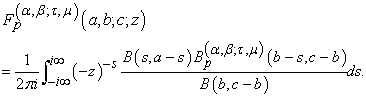(2.4)
2.4. The S-Generalized Gauss Hypergeometric Function Transform

We define the S-generalized Gauss hypergeometric transform by the following equation (see also a recent work  dealing with several new families of integral transforms):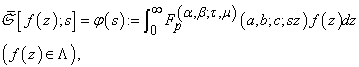(2.5)

where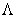denotes the class of functions for which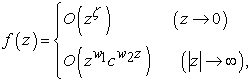(2.6)

provided that the existence conditions in (1.1) for the S-generalized Gauss hypergeometric functionare satisfied and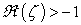and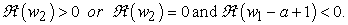(2.7)
2.5. Special Cases

In this section, we give three special cases of our integral transform defined by (2.5).

2.5.1. Generalize Gauss Hypergeometric Function Transform

If we putin (2.5), the transform in (2.5) reduces to the generalized Gauss hypergeometric function transform given by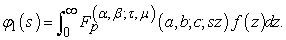(2.8)

2.5.2. Extension of the Generalized Gauss Hypergeometric Function Transform

By taking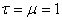in (2.8), we get the following extension of the generalized Gauss hypergeometric function transform: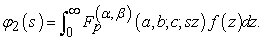(2.9)

Moreover, if we takein (2.9), it reduces to the extended Gauss hypergeometric function transform given below: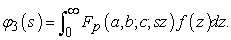(2.10)

if we set p = 0 in the integral transforms defined by (2.8), (2.9) and (2.10), we easily get the Gauss hypergeometric transform (see, for details, ).

2.6. Inversion Formula for the S-Generalized Gauss Hypergeometric Function Transform

Theorem 3. If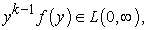the function f(y) is of bounded variation in the neighborhood of the point y = z, and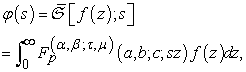(2.11)

then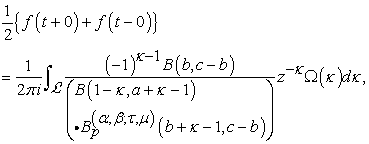(2.12)

where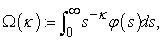(2.13)

provided that existence conditions for the S-generalized Gauss hypergeometric functiongiven by (1.1) are satisfied, the S-generalized Gauss hypergeometric function transform of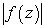exists, and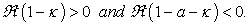Proof. In order to prove the inversion formula (2.12), we substitute the value of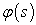from (2.11) into the right-hand side of (2.13). We thus find that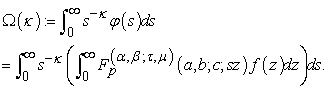(2.14)

Upon interchanging the order of the z- and the s-integrals in (2.14) (which is permissible under the given conditions), if we evaluate the s-integral by using (2.3), we obtain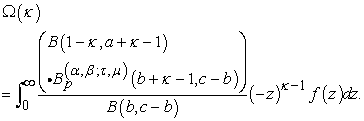(2.15)

Finally, by applying the Mellin Inversion Formula to the above integral (2.15), we get the desired result (2.12) after a little simplification.

### 3. Concluding Remarks and Observations

In our present investigation, we have further studied the S-generalized Gauss hypergeometric function: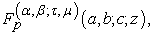which was recently introduced by Srivastava et al. . In the course of our study, we have presented an integral representation, the Mellin transform and a complex integral representation of the S-generalized Gauss hypergeometric function. We have also introduced a new integral transform whose kernel is the S-generalized Gauss hypergeometric function and pointed out its three special cases which are also believed to be new. Furthermore, we have specified that the well-known Gauss hypergeometric function transform follows as a simple special case of our integral transforms. Finally, we have established an inversion formula for the integral transform which we have introduced in this investigation.

### References

  M. A. Chaudhry, A. Qadir, H. M. Srivastava and R. B. Paris, Extended hypergeometric and conuentm hypergeometric functions, Appl. Math. Comput. 159 (2004), 589-602.In article View Article  L. Debnath and D. Bhatta, Integral Transforms and Their Applications, Third edition, Chapman and Hall (CRC Press), Taylor and Francis Group, London and New York, 2014.In article  S.-D. Lin, H. M. Srivastava and M.-M. Wong, Some applications of Srivastava's theorem involving a certain family of generalized and extended hypergeometric polynomials, Filomat 29 (2015), 1811-1819.In article View Article  S.-D. Lin, H. M. Srivastava and J.-C. Yao, Some classes of generating relations associated with a family of the generalized Gauss type hypergeometric functions, Appl. Math. Inform. Sci. 9 (2015), 1731-1738.In article  E. Özergin, Some Properties of Hypergeometric Functions, Ph.D. Thesis, Eastern Mediterranean University, Gazimağusa, North Cyprus, Turkey, 2011.In article  E. Özergin, M. A. Özarslan and A. Altın, Extension of gamma, beta and hypergeometric functions, J. Comput. Appl. Math. 235 (2011), 4601-4610.In article View Article  R. K. Parmar, A new generalization of Gamma, Beta, hypergeometric and conuent hypergeometric functions, Matematiche (Catania) 69 (2013), 33-52.In article  H. M. Srivastava, P. Agarwal and S. Jain, Generating functions for the generalized Gauss hypergeometric functions, Appl. Math. Comput. 247 (2014), 348-352.In article View Article  H. M. Srivastava, M. A. Chaudhry and R. P. Agarwal, The incomplete Pochhammer symbols and their applications to hypergeometric and related functions, Integral Transforms Spec. Funct. 23 (2012), 659-683.In article View Article  H. M. Srivastava and J. Choi, Seires Associated with the Zeta and Related Functions, Kluwer Academic Publishers, Dordrechet, Boston and London, 2001.In article  H. M. Srivastava and J. Choi, Zeta and q-Zeta Functions and Associated Series and Integrals, Elsevier Science Publishers, Amsterdam, London and New York, 2012.In article  H. M. Srivastava, K. C. Gupta and S. P. Goyal, The H-Functions of One and Two Variables with Applications, South Asian Publishers, New Delhi and Madras, 1982.In article  H. M. Srivastava and P. W. Karlsson, Multiple Gaussian Hypergeometric Series, Halsted Press (Ellis Horwood Limited, Chichester), John Wiley and Sons, New York, Chichester, Brisbane and Toronto, 1985.In article  H. M. Srivastava, M.-J. Luo, and R. K. Raina, A new integral transform and its applications, Acta Math. Sci. Ser. B Engl. Ed. 35 (2015), 1386-1400.In article View Article  H. M. Srivastava and H. L. Manocha, A Treatise on Generating Functions, Halsted Press (Ellis Horwood Limited, Chichester), John Wiley and Sons, New York, Chichester, Brisbane and Toronto, 1984.In article